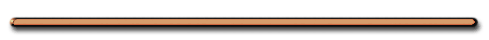AP Physics B B

Try this at home!

Mindpaint Channel

IV. Motion Equations for Problem Solving

When an object is moving at a constant acceleration we can use the following equations
to find motion informationA. Constant accel. Equations

(see Reference Table)

1. Average Velocity

As AP students you will need to memorize
all the constant accel. motion equations

V = ?

or

V = ?

V = (Vi + Vf)/2

or

V = Δd/Δt

2. Acceleration?

a = (Vf - Vi)/t

Cross multiply & Solve

at = (Vf - Vi)

 Vf = Vi + at3. Displacement:

 Δd = Vit + .... ?

 Δd = Vit +1/2at24. Final Velocity?

Vf2 = ....

Vf2 = Vi2 + 2aΔd